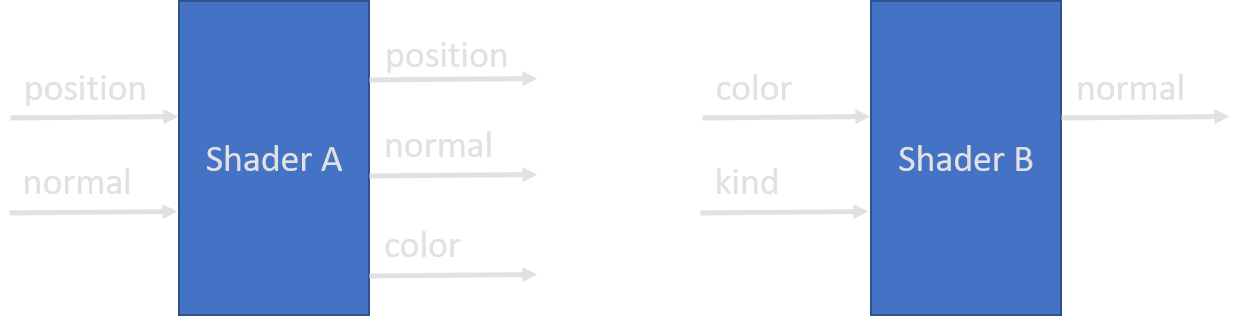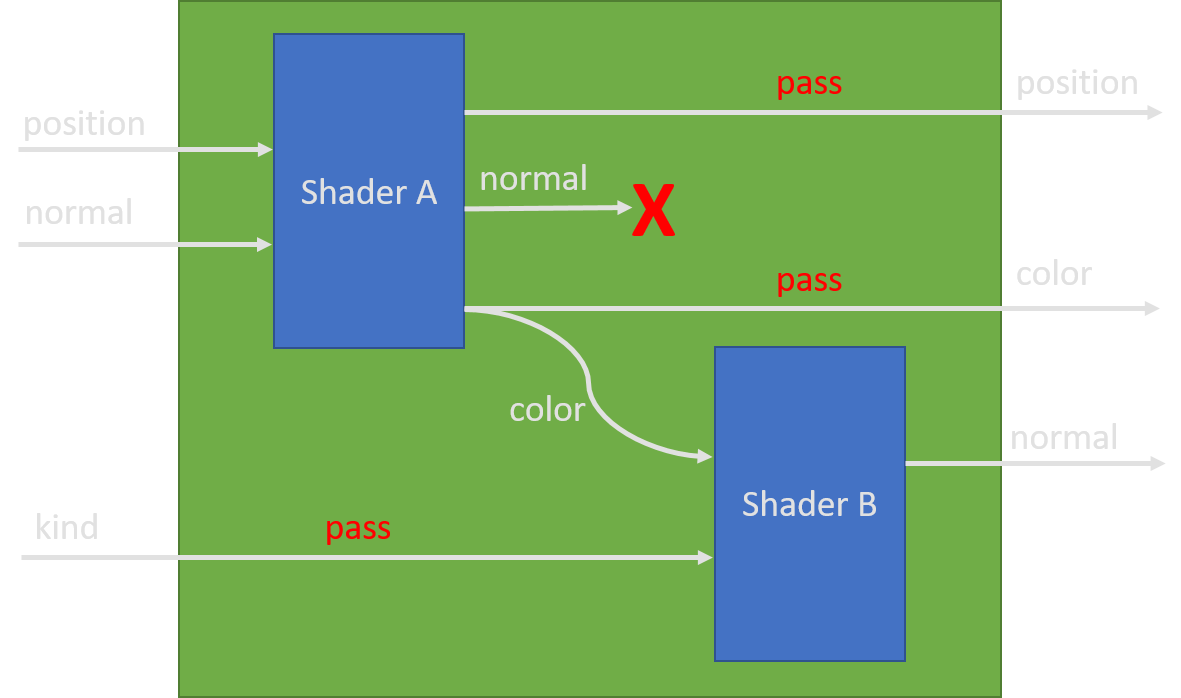# Composition

## Semantics

Since effect composition is rather complicated we'd like to elaborate its workings using pseudo code here.

From a high level perspective the GLSL/HLSL shader stages can be seen as functions

 ```1: 2: 3: 4: ``` ``````type VertexShader = Vertex -> Vertex type TessellationShader = Primitive -> seq> type GeometryShader = Primitive -> seq> type FragmentShader = Fragment -> Fragment ``````

As we see the first three stages (Vertex, Geometry and Tessellation) work on spatial vertices and the fragment shader operates on per-fragment data.

lets define some compose operators for these pseudo-shaders defining the composition semantics.

 ``` 1: 2: 3: 4: 5: 6: 7: 8: 9: 10: 11: 12: 13: 14: 15: 16: 17: 18: 19: ``` ``````let composeVertexVertex (l : VertexShader) (r : VertexShader) : VertexShader = // simply apply the right shader to the outputs of the left one l >> r let composeTessellationVertex (l : TessellationShader) (r : VertexShader) : TessellationShader = // apply the VertexShader to all vertice of all outputs of the TessellationShader l >> Seq.map (Primitive.map r) let composeGeometryVertex (l : GeometryShader) (r : VertexShader) : GeometryShader = // apply the VertexShader to all vertice of all outputs of the GeometryShader l >> Seq.map (Primitive.map r) let composeGeometryGeometry (l : GeometryShader) (r : GeometryShader) : GeometryShader = // apply the right shader to all primitives produced by the left one l >> Seq.collect r let composeFragmentFragment (l : FragmentShader) (r : FragmentShader) : FragmentShader = // simply apply the right shader to the outputs of the left one l >> r ``````

Based on these compositions we can now define compositions for tagged Shaders which will be helpful when implementing effect compositions below.

 ``` 1: 2: 3: 4: 5: 6: 7: 8: 9: 10: 11: 12: 13: 14: ``` ``````type Shader = | Vertex of VertexShader | Tessellation of TessellationShader | Geometry of GeometryShader | Fragment of FragmentShader let composeShader (l : Shader) (r : Shader) = match l, r with | Vertex l, Vertex r -> composeVertexVertex l r |> Vertex | Tessellation l, Vertex r -> composeTessellationVertex l r |> Tessellation | Geometry l, Vertex r -> composeGeometryVertex l r |> Geometry | Geometry l, Geometry r -> composeGeometryGeometry l r |> Geometry | Fragment l, Fragment r -> composeFragmentFragment l r |> Fragment | _ -> failwith "composition not possible" ``````

Since Vertex, Tessellation and Geometry work on primitives (or vertices) we refer to them as `PrimitiveShader`.

With these tools at hand we can now define the effect composition operator.

 ``` 1: 2: 3: 4: 5: 6: 7: 8: 9: 10: 11: 12: 13: 14: 15: 16: 17: 18: 19: 20: 21: 22: 23: 24: 25: 26: 27: 28: 29: 30: 31: 32: 33: 34: 35: 36: 37: 38: 39: 40: 41: 42: 43: 44: 45: 46: 47: 48: 49: 50: 51: 52: 53: 54: 55: 56: 57: 58: 59: 60: 61: 62: 63: 64: 65: ``` ``````type PrimitiveShader = { vertex : Option tessellation : Option geometry : Option } type Effect = { primitive : PrimitiveShader fragment : Option } let composePrimitive (l : PrimitiveShader) (r : PrimitiveShader) : PrimitiveShader = // apply all shaders in the order // [l.vertex; l.tessellation; l.geometry; r.vertex; r.tessellation; r.geometry] // using the compose functions defined above and fail if an impossible constellation // arises (e.g. composeTessellationTessellation would be needed) let shaders (p : PrimitiveShader) = [ match p.vertex with | Some s -> yield Vertex s | None -> () match p.tessellation with | Some s -> yield Tessellation s | None -> () match p.geometry with | Some s -> yield Geometry s | None -> () ] let withShader (s : Shader) (p : PrimitiveShader) = match s with | Geometry gs -> { p with geometry = Some gs } | Tessellation ts -> { p with tessellation = Some ts } | Vertex vs -> { p with vertex = Some vs } | _ -> p match l with | { geometry = Some lg } -> l |> withShader (r |> shaders |> List.fold composeShader (Geometry lg)) | { tessellation = Some lt } -> l |> withShader (r |> shaders |> List.fold composeShader (Tessellation lt)) | { vertex = Some lv } -> l |> withShader (r |> shaders |> List.fold composeShader (Vertex lv)) | _ -> r [] module Effect = let empty = { primitive = { vertex = None; tessellation = None; geometry = None } fragment = None } let compose2 (l : Effect) (r : Effect) = { primitive = composePrimitive l.primitive r.primitive fragment = match l.fragment, r.fragment with | o, None | None, o -> o | Some l, Some r -> Some (composeFragmentFragment l r) } ``````

As we see here `Effect.compose2` treats primitive- and fragment-shaders separately and ensures ordering only inside the primitive-stage. Arguably the composition could simply fail if called like `Effect.compose2 { fragment = Some f } { primitive = <non-empty> }` but we decided to keep it as flexible as possible.

### Neutral Element

`Effect.empty` poses as the neutral element for `Effect.compose2` which can easily be seen by looking at the code. Therefore `Effect.compose2 Effect.empty a = a` and `Effect.compose2 a Effect.empty = a` hold.

### Associativity

Since `Effect.compose2` resembles function composition `(>>)` it obviously inherits its associativity. Therefore `Effect.compose2 a (Effect.compose2 b c) = Effect.compose2 (Effect.compose2 a b) c` holds.

Note that it is not commutative which means `Effect.compose2 a b <> Effect.compose2 b a` and also not idempotent meaning `Effect.compose a a <> a`.

These properties motivate the combinator

 ```1: 2: ``` `````` let compose (effects : seq) : Effect = effects |> Seq.fold compose2 empty ``````

## Realworld

Real FShade Effects do not provide simple functions but instead contain Shaders for some of the stages. Since these Shaders are not required to have matching inputs/outputs implementing the composition gets a bit more tedious.

From a high-level point of view FShade's Shaders can be seen as modules with an arbitrary number of inputs and outputs which are semantically tagged using a name.FShade composes these in the following wayAs you see in the illustration we need to be able to pass values through shaders which is rather simple for the vertex/fragment stages but hard for geometry and tessellation.

In order to pass values through tessellation shaders we simply interpolate them using the given domain when possible. (more on that in Tessellation)

For geometry shaders the passing cannot be implemented unless the input-primitives are `Point<'a>` or the user provides hints for the passing. This is done via the `[<SourceVertexIndex>]` attribute which is discussed in detail in Geometry.

namespace Microsoft
namespace Microsoft.FSharp
namespace Microsoft.FSharp.Quotations
namespace Aardvark
namespace Aardvark.Base
Multiple items
union case Vertex.Vertex: Vertex

--------------------
type Vertex = | Vertex
Multiple items
union case Fragment.Fragment: Fragment

--------------------
type Fragment = | Fragment
type Primitive<'a> = 'a list
type 'T list = List<'T>
val map : mapping:('a -> 'b) -> p:Primitive<'a> -> Primitive<'b>
val mapping : ('a -> 'b)
val p : Primitive<'a>
Multiple items
module List

--------------------
module List

from Aardvark.Base.Prelude

--------------------
module List

from Aardvark.Base.Predefined Lenses

--------------------
module List

from Aardvark.Base.Arrays

--------------------
module List

from Microsoft.FSharp.Collections

--------------------
type List<'T> =
| ( [] )
| ( :: ) of Head: 'T * Tail: 'T list
interface IEnumerable
interface IEnumerable<'T>
member GetSlice : startIndex:int option * endIndex:int option -> 'T list
member IsEmpty : bool
member Item : index:int -> 'T with get
member Length : int
member Tail : 'T list
static member Cons : head:'T * tail:'T list -> 'T list
static member Empty : 'T list
val map : mapping:('T -> 'U) -> list:'T list -> 'U list
type VertexShader = Vertex -> Vertex
type TessellationShader = Primitive<Vertex> -> seq<Primitive<Vertex>>
Multiple items
module Primitive

from Composition

--------------------
type Primitive<'a> = 'a list
Multiple items
val seq : sequence:seq<'T> -> seq<'T>

--------------------
type seq<'T> = System.Collections.Generic.IEnumerable<'T>
type GeometryShader = Primitive<Vertex> -> seq<Primitive<Vertex>>
type FragmentShader = Fragment -> Fragment
Multiple items
active recognizer Seq: Expr -> Expr list option

--------------------
module Seq

from Aardvark.Base.Prelude

--------------------
module Seq

from Aardvark.Base.Arrays

--------------------
module Seq

from Microsoft.FSharp.Collections
val map : mapping:('T -> 'U) -> source:seq<'T> -> seq<'U>
val collect : mapping:('T -> #seq<'U>) -> source:seq<'T> -> seq<'U>
Multiple items

--------------------
Multiple items

--------------------
type Vertex = | Vertex
Multiple items

--------------------
module Geometry

from Aardvark.Base

--------------------
type Geometry =
{vertexAttributes: Map<Symbol,IMod<IBuffer>>;
indices: Option<BufferView>;
uniforms: Map<string,IMod>;
call: IMod<DrawCallInfo list>;}
Multiple items

--------------------
type Fragment = | Fragment
val failwith : message:string -> 'T
Multiple items
module Option

--------------------
module Option

from Aardvark.Base.Prelude

--------------------
module Option

from Microsoft.FSharp.Core
Multiple items
module Effect

--------------------
type Effect =
union case Option.Some: Value: 'T -> Option<'T>
union case Option.None: Option<'T>
val tessellation : TessBuilder
val vertex : VertexBuilder
val fold : folder:('State -> 'T -> 'State) -> state:'State -> list:'T list -> 'State
Multiple items
type CompilationRepresentationAttribute =
inherit Attribute
new : flags:CompilationRepresentationFlags -> CompilationRepresentationAttribute
member Flags : CompilationRepresentationFlags

--------------------
new : flags:CompilationRepresentationFlags -> CompilationRepresentationAttribute
type CompilationRepresentationFlags =
| None = 0
| Static = 1
| Instance = 2
| ModuleSuffix = 4
| UseNullAsTrueValue = 8
| Event = 16
CompilationRepresentationFlags.ModuleSuffix: CompilationRepresentationFlags = 4
Multiple items
module Effect

from Composition

--------------------
module Effect

--------------------
type Effect =